Courses

# Test: Computation Operations

## 20 Questions MCQ Test Mathematics for Class 5 (V) - CBSE and NCERT Curriculum | Test: Computation Operations

Description
This mock test of Test: Computation Operations for Class 5 helps you for every Class 5 entrance exam. This contains 20 Multiple Choice Questions for Class 5 Test: Computation Operations (mcq) to study with solutions a complete question bank. The solved questions answers in this Test: Computation Operations quiz give you a good mix of easy questions and tough questions. Class 5 students definitely take this Test: Computation Operations exercise for a better result in the exam. You can find other Test: Computation Operations extra questions, long questions & short questions for Class 5 on EduRev as well by searching above.
QUESTION: 1

### Find the value of MMDCCCLX - MMDCCLIII

Solution:

MMDCCCLX-MMDCCLIII = 2860 − 2753 = 107 = CVII

QUESTION: 2

### Fathom is a unit once used by sailors to measure the depth of water, if a sunken ship was located underwater at 240 feet, which expression would describe the location of the ship in fathoms? 1 fathom = 6 feet

Solution:

We have, 1 fathom = 6 feet
1 feet = 1/6 fathom Ship was located underwater at 240 feet.
∴ Location of ship underwater in fathoms = 240-6

QUESTION: 3

### Which of the following options hold? Statement 1: if 7 friends divide 76895 coloured beads amongst themselves, then each will get 10984 coloured beads. Statement 2: If 16 families went on a trip and pay 216352 in total, then each family will pay 13522.

Solution:

Statement-1: If 7 friends divide 76895 beads, each friend will get (76895÷7) beads i.e., 10985 beads.
So, statement-1 is false. Statement-2: Each family will pay (216352−16)= 13522 So, statement-2 is true.

QUESTION: 4

Which of the following is NOT true?

Solution:
QUESTION: 5

Which of the following options makes the given expression true?

990064 + 420349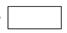912345 + 521935

Solution: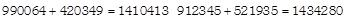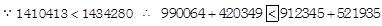QUESTION: 6

A factory produces 900640 tyres in the month of May. Out of these, 6782 tyres were found defective in the quality check. How many tyres were not defective?

Solution:

Total number of tyres produced = 900640 Number of defective tyres = 6782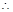Number of tyres which are not defective = 900640 - 6782 = 893858

QUESTION: 7

What should be subtracted from the greatest 7 digit number to get 65,43,210 ?

Solution:

Let x be the number which should be subtracted from greatest 7 digit number 9999999 to get 6543210.
Then 9999999−x=6543210⟹x=9999999−6543210=34567899
The required number is 3456789

QUESTION: 8

Mohit baked 2000 cookies. He sold 600 of them and gave the rest equally to 20 of his friends. How many cookies did each of his friend receive?

Solution:

Number of cookies baked = 2000
Number of cookies sold = 600
Remaining number of cookies = 2000 − 600 = 1400
Number of friends == 20
∴ Number of cookies each friend will receive = 1400/2 = 70

QUESTION: 9

The product of 214 and a number is X. Taking 49 away from X gives 1449. Find the number.

Solution:

According to question,
we have x - 49 = 1449
⇒ x = 1449 + 49 = 1498
Now,
required number = 1498/214 = 7

QUESTION: 10

The value of 14 × 7 + 36/4 + 14 =_____.

Solution:
QUESTION: 11

Highest common factor of 42 and 162 is______.

Solution: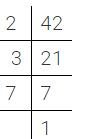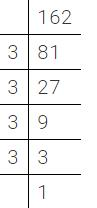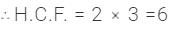QUESTION: 12

A contractor sent 7695940 bricks for the construction of 70 chambers. If an equal number of bricks were required for each chamber, how many bricks were used for each chamber?

Solution:

Total number of bricks = 7695940
Number of chambers = 70
So, number of bricks used for each chamber 7695940/70 = 109942

QUESTION: 13

Least common multiple of 8, 12 and 20 is

Solution: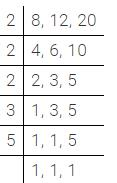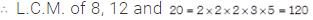QUESTION: 14

In a school, there are 704 desks to be placed in 22 classrooms. If the same number of desks are placed in each classroom, how many desks will be there in each room?

Solution:

Total number of desks = 704
Number of classrooms = 22
∴ Number of desks in each classroom = 704/22 = 32

QUESTION: 15

Subtract the sum of 7523625 and 2234374 from 61276343.

Solution:

Sum of 7523625 and 2234374 = 7523625 + 2234374  = 9757999
∴ Required difference = 61276343 - 9757999 = 1518344

QUESTION: 16

Which of these could be solved by using the sentence 'A - 5'?

Solution:

(A) Age of Ishan = 5A (B) Age of Tarun = A - 5 (C) Age of Aman = 5A (D) Age of Geet = A + 5

QUESTION: 17

The quotient when 222816 is divided by 24 Is ________.

Solution:
QUESTION: 18

Maruti Motors produces 127695 cars in the year 2007; 98895 cars in the year 2008 and 305789 cars in the year 2009. What was the total number of cars produced in three years altogether?

Solution:
QUESTION: 19

In a certain state, 5261989 students were enrolled in various schools. Of these, 1965233 students enrolled in primary school, 2006756 students enrolled in high school and the rest in middle school. How many ' students enrolled in middle school?

Solution:

Total number of students enrolled = 5261989
Number of students enrolled in primary school and high school altogether 1965233 + 2006756 = 3971989
∴ Number of students enrolled in middle school = 5261989 - 3971989 = 1290000

QUESTION: 20

Farmer Gopal packed an equal number of oranges into each of the 15 baskets. If each basket contained 85 oranges, how ' many oranges did he pack?

Solution:

Total number of baskets =15
Number of oranges in each basket =85
Total number of oranges packed = 15 ×85 = 1275